Journal of Vibroengineering

Published: 31 December 2018

# Vibration control of a hydrostatic bearing using magnetorheological elastomer shell bearing

Noureddine Chikh1
Salah Aguib2
Toufik Djedid3
1, 2, 3, 4Dynamic Motors and Vibroacoustic Laboratory, M’Hamed Bougara University of Boumerdes, Algeria
5Laboratoire QUARTZ(EA7393), Supmeca, 3 rue de Fernand Hainaut, 93407 Saint-Ouen Cedex, France
Corresponding Author:
Noureddine Chikh
Corresponding Author:
Salah Aguib
Views 298

#### Abstract

In rotating machines, random movements or sliding movements of the rotor in its housing can produce undesirable phenomena for some parts. To solve this problem, a new hydrostatic bearing with an intelligent magnetorheological elastomer sell bearing has been designed to control the undesirable vibrations of rigid rotors. The different effects of the influence parameters on the vibratory behavior of the sell bearing are calculated numerically using Abaqus software, and the results found are encouraging.

## 1. Introduction

The technological evolution of rotating machinery requires high performance operating conditions in terms of power, rotational speed and load capacity; for example, the critical speed of the rotor at the beginning of operating is a harmful factor limiting the life of the mechanism .

The main object of our work is to design an intelligent hydrostatic bearing with magnetorheological elastomer sell bearing, to control the vibrations of rigid rotors during the passage of critical speeds, by the control of the damping and the rigidity of the bearings.

## 2. Bearing model with MRE shell bearing

The bearings used in rotating machines (turbines, centrifugal pumps) generally comprise bearings whose main function is to ensure proper operating and to better control the vibration of the bearing Fig. 1, as well as the physical model supported by two bearings is represented by Fig. 2.

Fig. 1Hydrostatic bearing with magnetorheological elastomer shell bearing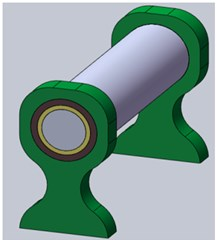a)b)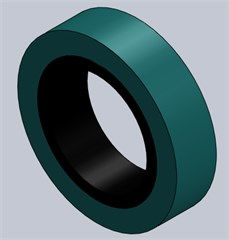c)

Fig. 2Physical model supported by two bearings with magnetorheological elastomer shell bearing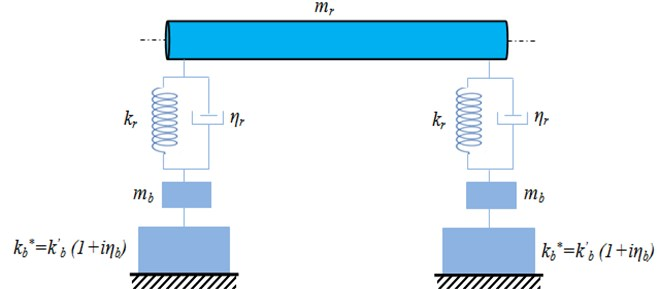## 3. Mathematical modeling of vibrations

### 3.1. Modeling of the rotor behavior

The shaft is modeled by a rotating Timoshenko beam. The general formulation of the kinetic  and the deformation energies of the shaft integrating these formulas on the length of the shaft, it comes:

1
${E}_{c}=\frac{{\rho }_{r}}{2}\underset{0}{\overset{l}{\int }}\left[{A}_{r}\left({\left(\frac{\partial u}{\partial t}\right)}^{2}+{\left(\frac{\partial v}{\partial t}\right)}^{2}+{\left(\frac{\partial w}{\partial t}\right)}^{2}\right)+{I}_{r0}\left({\left(\frac{\partial {\theta }_{u}}{\partial t}\right)}^{2}+{\left(\frac{\partial {\theta }_{v}}{\partial t}\right)}^{2}+{I}_{rp}\left({\left(\frac{\partial \phi }{\partial t}+\frac{\partial \beta }{\partial t}\right)}^{2}+2\left(\frac{\partial \phi }{\partial t}+\frac{\partial \beta }{\partial t}\right)\right)\frac{\partial {\theta }_{u}}{\partial t}\frac{\partial {\theta }_{v}}{\partial t}\right)\right]dz.$

The deformation energy of a rotating Timoshenko beam  is equal to:

2
${E}_{d}=\frac{1}{2}\underset{0}{\overset{l}{\int }}\left[{E}_{r}{A}_{r}{\left(\frac{\partial w}{\partial z}\right)}^{2}+{E}_{r}{I}_{rx}\left({\left(\frac{\partial {\theta }_{u}}{\partial z}\right)}^{2}+{\left(\frac{\partial {\theta }_{v}}{\partial z}\right)}^{2}\right){G}_{r}{I}_{rp}{\left(\frac{\partial \beta }{\partial z}\right)}^{2}+k{G}_{r}{A}_{r}\left({\left(\frac{\partial u}{\partial z}+{\theta }_{v}\right)}^{2}+{\left(\frac{\partial v}{\partial z}+{\theta }_{u}\right)}^{2}\right)\right]dz,$

where $k$ is the correction factor for shear stiffness.

### 3.2. Modeling the behavior of the shell bearing

The damped vibratory movement of the MRE shell bearing is modeled by the system given in Fig. 3.

Fig. 3The MRE shell bearing model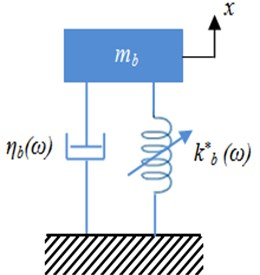The equation of movement of the MRE shell bearing of Fig. 3 is given from the second law of motion, $F=ma$ , in which $F$ is equivalent to the sum of the restoring force $-{k}^{*}x$ and the damping force ${k}^{*}x$. In this last expression, $\eta$ represents a positive damping which is adjustable by the application of a magnetic field. So, we get:

3
$-{k}_{b}^{\mathrm{*}}x-{\eta }_{b}\frac{dx}{dt}={m}_{b}\frac{{d}^{2}x}{d{t}^{2}},$
4
${m}_{b}\frac{{d}^{2}x}{d{t}^{2}}+{k}_{b}^{\mathrm{*}}x+{\eta }_{b}\frac{dx}{dt}=0.$

The solution of this differential equation corresponds to:

5
$x=A{e}^{-{\eta }_{b}t/2{m}_{b}}\mathrm{c}\mathrm{o}\mathrm{s}\left(\omega t+\varphi \right).$

In the case of a magnetorheological elastomer, the complex rigidity is given by:

6
${k}_{b}^{*}\left(\omega \right)={k}_{b}^{\text{'}}\left(\omega \right)+i{k}_{b}^{\text{'}\text{'}}\left(\omega \right)={k}_{b}^{\text{'}}\left(1+{\eta }_{b}\left(\omega \right)\right)$

then:

7
$\omega =2\pi f=\sqrt{\frac{{k}_{b}^{\text{'}}\left(1+{\eta }_{b}\right)}{{m}_{b}}+{\left(\frac{{\eta }_{b}}{2{m}_{b}}\right)}^{2}}.$

It is also possible to define a complex viscosity as follows:

8
$\sigma ={\eta }_{b}^{*}\left(\omega \right)\stackrel{˙}{\gamma }=\left({\eta }^{\text{'}}-i{\eta }_{b}^{\text{'}\text{'}}\right)\stackrel{˙}{\gamma },$

with:

9
${\eta }_{b}^{\text{'}}=\frac{{k}^{\text{'}\text{'}}}{\omega },$
10
${\eta }_{b}^{\text{'}\text{'}}=\frac{{k}^{\text{'}}}{\omega },$

where ${\eta }_{b}^{\text{'}}$ and ${\eta }_{b}^{\text{'}\text{'}}$ are associated with the loss modulus and the conservation modulus respectively.

### 3.3. Modeling of the magnetorheological elastomer

For small displacements and relatively small times t, the response of a viscoelastic material is dominantly elastic for constant strain rates, and that for larger values of $t$, the response is dominantly or entirely viscous.

The Maxwell model can accurately describe creep behavior in magnetorheological elastomer with small displacements. This model represents a viscoelastic material with several parallel branches such as a spring and a dashpot in parallel and is particularly accurate in predicting creep behavior.

Prony series parameters $g$, $k$, and $\tau$ were needed to characterize the creep displacement behavior in Abaqus. Shear and bulk moduli were then calculated using these parameters (Keenan) in order to compute the analytical solution:

11
${g}_{R}\left(t\right)=1-{\sum }_{i}^{n}{g}_{i}\left(1-{e}^{-\frac{t}{\tau }}\right),$
12
${k}_{R}\left(t\right)=1-{\sum }_{i}^{n}{k}_{i}\left(1-{e}^{-\frac{t}{\tau }}\right).$

## 4. Elaboration and experimental analysis of MRE

### 4.1. Steps elaboration and mechanical characterization

The rheological parameters of the MRE shell bearing as a function of the magnetic field are determined experimentally using the MetraviB DMA + 450 viscoanalyser Fig. 4(b) (PMC Laboratory, Nice, France). The elastomer is prepared (Fig. 4(a)) by the following steps:

Mixing the silicone oil and the RTV141A polymer in a bowl and proceed with a manual mixing during 10 min to obtain an elastomer gel with good homogenization. A second bowl containing a quantity of iron particles of micrometric size to loading the elastomer is prepared.

Fig. 4a) Prepared elastomer, b) MetraviB DMA + 450 Viscoanalyser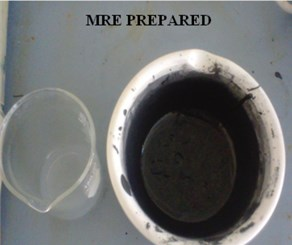a)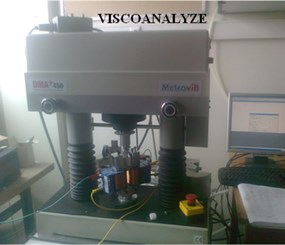b)

A quantity of this gel obtained by silicone and RTV141A is mixed during 30 min with a quantity of iron particles until obtaining a homogeneous paw. By this process, an elastomer loaded with 30 % of ferromagnetic particles is elaborated.

The degassing of the obtained paw under vacuum during 10 min to eliminate air bubbles in filtrated during the mixing is performed in order to have a healthy structure for the experimentation. The obtained elastomer is hermetically pre- served at low temperature.

The specimen has a rectangular shape of 30 mm of length, 28 mm of width, and 2 mm of thickness and loaded with 40 % of ferromagnetic particles of its total volume. The values of the storage and loss modulus obtained with and without the magnetic field are given in Table 1 .

Table 1Experimental data of shear moduli G' and G''

 $I=$ 0 A $I=$ 0.5 A $I=$ 1.2 A $I=$ 2 A ${G}^{\text{'}}$ (MPa) ${G}^{\text{'}\text{'}}$ (MPa) ${G}^{\text{'}}$ (MPa) ${G}^{\text{'}\text{'}}$ (MPa) ${G}^{\text{'}}$ (MPa) ${G}^{\text{'}\text{'}}$ (MPa) ${G}^{\text{'}}$ (MPa) ${G}^{\text{'}\text{'}}$ (MPa) 1.8328 0.3236 2.1461 0.7036 3.1527 1.0334 2.9660 0.7248 1.2847 0.3148 1.5403 0.5410 2.1824 0.7340 2.5806 0.6335 1.01643 0.2495 1.2133 0.4019 1.6262 0.5323 2.3134 0.5763 0.8186 0.2200 0.8716 0.2979 1.1262 0.3482 1.7035 0.4636 0.6805 0.1895 0.7658 0.2770 0.8908 0.2947 1.3160 0.3595 0.6197 0.1521 0.7143 0.2317 0.7546 0.3206 1.1240 0.3136

## 5. Numerical simulation of shell bearing

To deal with the problem of reducing the amplitude of vibrations by the MRE shell bearing and the housing, we used a multi-body equivalent model in the Abaqus software (Fig. 5(a), (b)). The results of the meshing give 2476 elements for the shell bearing and 4805 for the housing.

Fig. 5Finite elements model of a bearing: a) without mesh, b) with mesh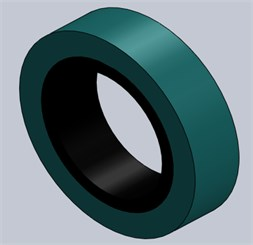a)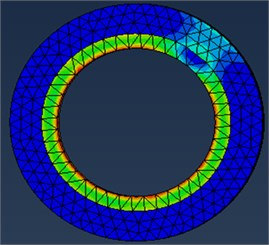b)

The geometric and mechanical parameters of the studied bearing are given in Table 2.

Table 2Geometric and mechanical parameters of the bearing

 Housing radius 25 mm Width of the housing 25 mm Thickness of the shell bearing 2 mm Elasticity modulus of the housing 199000 MPa Poisson’s ratio of the housing 0.29 Density of the housing 7800 kg/m3 Poisson’s ratio of the shell bearing 0.45 Density of the shell bearing 1100 kg/m3 Fluid supply pressure 0.1 MPa

### 5.1. Results and discussions

An implicit model using the Abaqus software is taken up using the six-branch Maxwell generalized rheological model and the Prony series to analyze the influence of the MRE shell bearing on the rotor vibratory behavior. After recalling the main mechanical and rheological characteristics of the different parts, a complete modeling of the bearing and housing by the finite element method using the Abaqus software which allow meshing each of the two parts by C3D10 parabolic tetrahedron elements to ensure the convergence Fig. 5. Nevertheless, the representation of the viscoelastic core by volume elements is intended to take into account the shear responsible for damping in this layer. The numerical results found of the various parameters as a function of the intensity of the magnetic field are given by the Table 3.

Table 3The numerical results found of the different parameters according to the intensity of the current

 $I=$ 0 A $I=$ 0.5 A $I=$ 1.2 A $I=$ 2 A $U$ (mm) $\eta$ $F$ (Hz) $U$ (mm) $\eta$ $F$ (Hz) $U$ (mm) $\eta$ $F$ (Hz) $U$ (mm) $\eta$ $F$ (Hz) 1.304 0.18 396.24 1.300 0.33 587.48 1.293 0.33 717.60 1.289 0.24 604.94 1.001 0.24 397.69 1.001 0.35 589.62 1.001 0.34 720.16 1.001 0.24 607.01 1.267 0.25 404.22 1.282 0.33 617.06 1.277 0.33 732.06 1.271 0.25 599.34 1.004 0.27 406.22 1.005 0.34 620.73 1.004 0.31 736.14 1.004 0.27 602.46 1.352 0.24 413.49 1.363 0.32 632.39 1.351 0.33 749.75 1.348 0.27 613.38

The displacement response corresponding to the first five vibration modes of the MRE shell bearing at 27°C and under a harmonic stress is shown in Fig. 6 as a function of different values of the current intensity. It is found that the amplitude is maximum and reaches critical values in the modes 1, 3 and 5. A low amplitude for modes 2 and 4. This observation is clearly shown in Fig. 7; the amplitude reaches critical values for odd modes and low amplitudes for even modes.

Fig. 6Variation of the amplitude as a function of the current intensity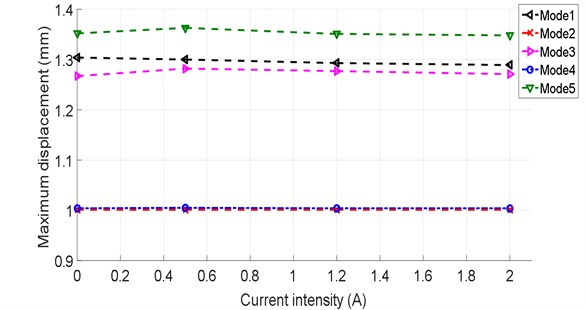Fig. 7 shows the evolution of maximum displacement of the shell bearing modeling using Abaqus, as a function of natural frequencies. We found surprisingly that the natural frequency increases with the increase of the current intensity. There is also a significant increase in the frequency between a zero current intensity and for current intensities ranging between 0.5 and 2 A which gives an increase of the order of 53 %. This is due to the effect of the electric field on the rigidity of the system.

This figure shows that the displacements of the rotor are reduced as a function of the increase of the magnetic field and this is due to the increase of the rigidity of the MRE shell bearing. As the frequency increases with the increase of the intensity of the magnetic field (that is to say as a function of the increase of the rigidity of the MRE shell bearing).

The results in Table 3 show us that the damping of the MRE shell bearing increases with the electric field. The variation of the damping rate is shown in Fig. 8, it can be seen that the damping rate of the shell bearing increases rapidly with the electric field for low values of current intensity, practically of up to 0.5 A, and then we notice a stabilization phase for intensity values varying from 0.5 to 1.2 A, and then gradually decreasing. This is due to the increase of the attractive force between the ferromagnetic particles due to the increase of the electric field. The damping rate increases by 45 % for the first phase (0 ≤ $I$ ≤ 0.5) A, which represents a significant increase. We can see an asymptotic increase in the damping rate for the second phase, which shows its limit.

Fig. 7Modes and frequencies of vibration according to the current intensity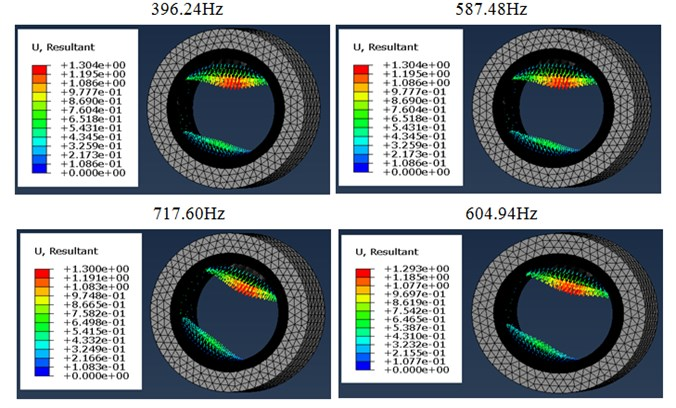a) 1st mode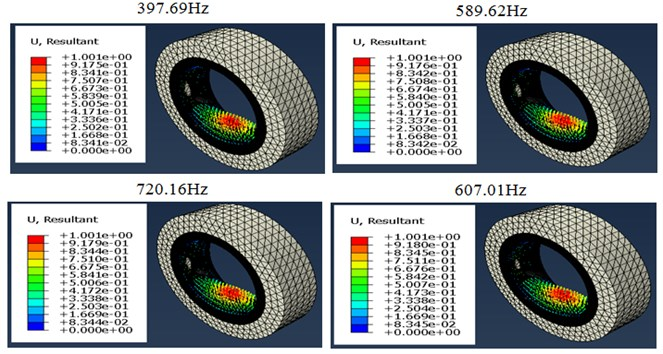b) 2nd mode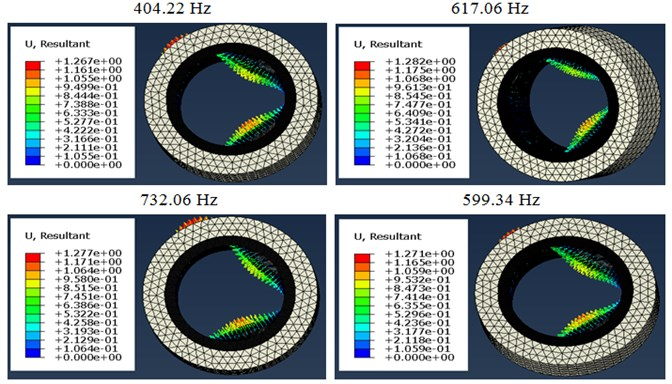c) 3rd mode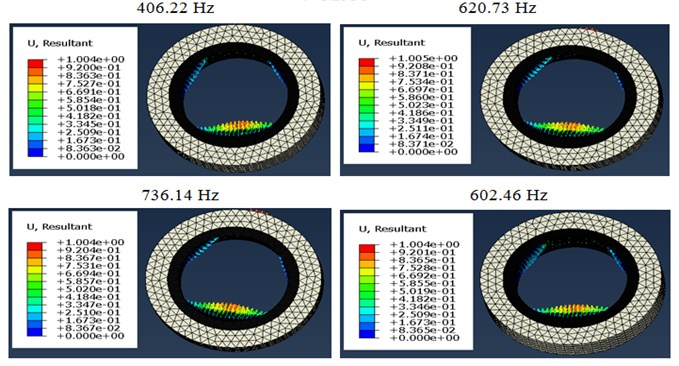d) 4th mode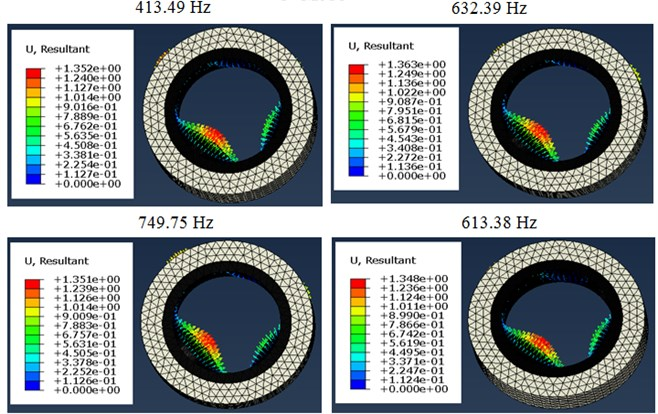e) 5th mode

Fig. 8Variation of the damping rate according to the current intensity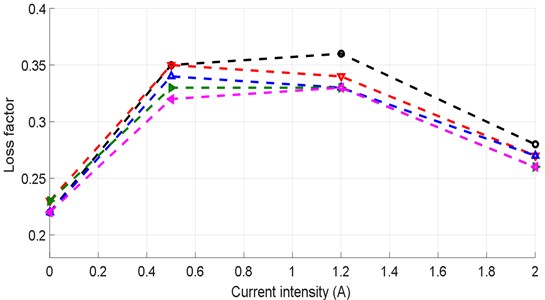The results of the identification of the mechanical behavior (Table 4) of the shell bearing as a function of the magnetic field intensity are presented in Fig. 9. It appears that the increase in the magnetic field intensity makes it possible to appreciably increase the stress and the deformation. We have found that in the presence of a magnetic field, the attractive force between the iron particles increases which gives a greater sliding movement, these two phenomena are the basis of increase of the stress and the deformation simultaneously.

Table 4Variation of the stresses vs. strain under different magnetic field intensities

 $B=$ 0 T $B=$ 0.15 T $B=$ 0.3 T $B=$ 0.45 T $\epsilon$ $\sigma$ (MPa) $\epsilon$ $\sigma$ (MPa) $\epsilon$ $\sigma$ (MPa) $\epsilon$ $\sigma$ (MPa) 2.822×10-5 2.957×10-3 2.131×10-5 3.022×10-3 1.990×10-5 3.053×10-3 1.929×10-5 3.084×10-3 6.373×10-5 3.326×10-3 4.460×10-5 3.399×10-3 4.027×10-5 3.435×10-3 3.783×10-5 3.470×10-3 9.925×10-5 3.696×10-3 6.789×10-5 3.777×10-3 6.063×10-5 3.816×10-3 5.637×10-5 3.855×10-3 1.348×10-4 4.066×10-3 9.188×10-5 4.155×10-3 8.100×10-5 4.198×10-3 7.491×10-5 4.241×10-3 1.703×10-4 4.435×10-3 1.145×10-4 4.532×10-3 1.014×10-4 4.580×10-3 9.345×10-5 4.626×10-3

Fig. 9Variation of the stress developed within the shell bearing according to the strain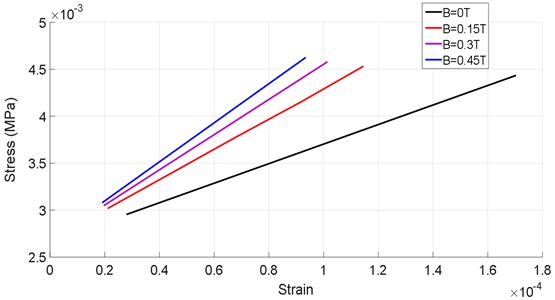Table 5Variation of the displacements vs to the strain under different the intensity of the magnetic field

 $B=$ 0 T $B=$ 0.15 T $B=$ 0.3 T $B=$ 0.45 T $U$ (mm) $\sigma$ (MPa) $U$ (mm) $\sigma$ (MPa) $U$ (mm) $\sigma$ (MPa) $U$ (mm) $\sigma$ (MPa) 3.540×10-4 4.435×10-3 2.363×10-4 4.532×10-3 2.086×10-4 4.580×10-3 1.917×10-4 4.626×10-3 6.606×10-4 4.066×10-3 4.424×10-4 4.155×10-3 3.911×10-4 4.198×10-3 3.599×10-4 4.241×10-3 9.672×10-4 3.696×10-3 6.485×10-4 3.777×10-3 5.735×10-4 3.816×10-3 5.281×10-4 3.855×10-3 1.274×10-3 3.326×10-3 8.546×10-4 3.399×10-3 7.560×10-4 3.435×10-3 6.963×10-4 3.470×10-3 1.580×10-3 2.975×10-3 1.061×10-3 3.022×10-3 9.385×10-4 3.053×10-3 8.645×10-4 3.084×10-3

Fig. 10Variation of the stress developed within the shell bearing according to the displacement of the rotor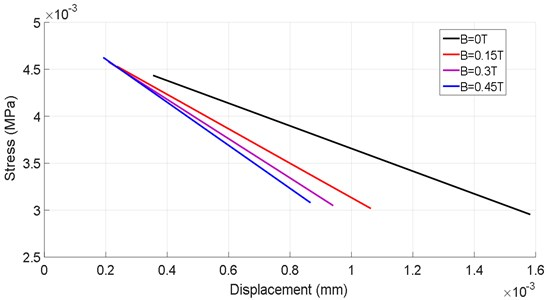The variation of the stress developed within the shell bearing as a function of the displacement (Table 5) of the rotor is shown in Fig. 10. There is a considerable decrease in rotor displacements with the increase of the magnetic field intensity, in the case of $B=$ 0 T, one has a displacement increasing from (0.38 to 1.5) .10-3 unit on the other hand in the case of $B=$ 0.45 T one has a displacement increasing from (0.18 to 0.83). 10-3 unit, thus with a rate of reduction great than 50 %.

## 6. Conclusions

In this work, a new hydrostatic bearing with magnetorheological elastomer shell bearing has been designed to control the undesirable vibrations of rigid rotors.

This system of active vibration control of rigid rotors using magnetorheological elastomer bearings has aroused great interest for engineers and researchers because it combines the advantages of active-passive control, and it does not need great external energy, it responded even with low electrical intensity.

The Maxwell model is used to better describe the rheological behavior of the magnetorheological elastomer using the Abaqus software. This model is based on experimental data. This model of MRE damper has been applied to a simplified system with one degree of freedom with complex rigidity. However, this model allowed us to realize numerically a system of active control of rotor vibrations. The results of the numerical simulation can be summarized as follows:

The magnetorheological elastomer shell bearing of a hydrostatic bearing was used as an effective control system to limit the vibration amplitude on the one hand and to reduce the transmitted force on the other hand. The application of the electric field has a significant influence on the damping rate at low current intensity, whereas the increase in intensity has no effect on the damping rate. The increase in the intensity of the electric field increases the rigidity, and therefore, the damping rate decreases beyond the stabilization intensity and this is due to the reduction of the dissipative modulus. To reduce the amplitude of the vibratory response of the excited rotor by an imbalance, it is sufficient to use an MRE shell bearing with a low intensity to obtain a high damping. Otherwise, the use of high electric field intensity is not necessary. On the other hand, the use of an MRE shell bearing with high electric field intensity is very effective in reducing the force transmitted to the base. When the rotor is operating near the critical speed, the electric field must not be high in order to obtain high damping.

#### Acknowledgements

Thanks to Laboratory of Condensed Matter Physics (LPMC) University of Nice – Sophia Antipolis – France, for providing various supports for this study. We are also grateful to Messrs George Bossis Research Director Emeritus and Dr. Kuzhir Pavel of LPMC, for their help.